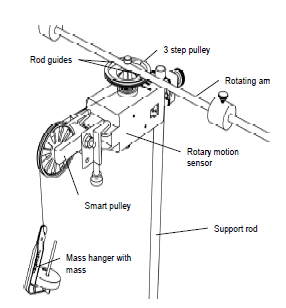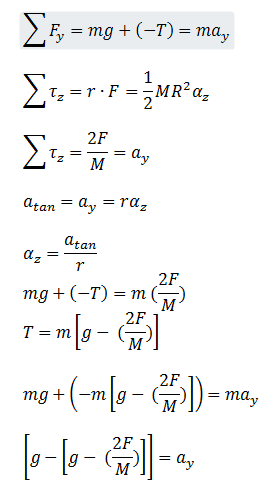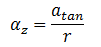# Moment of Inertia of pulley1. Homework Statement

In each question: you place mass m on the mass holder, and released the system from rest. The system includes the long rod with two point masses on it (see the picture next to the list of equipment).

Since this is a rotational and translational motion, I set up two equations: sum of the force and torque

unknown will be angular acceleration and moment of inertia.

## Homework Equations## The Attempt at a Solution

Sorry. I guess I put my solution in the above.
a)
if you increase m, the mass on the mass holder, what happens to the following quantities:
- α, the angular acceleration of the pulley.
- I, the moment of inertia of the pulleys-plus-rod system.

The change of mass in the holder won't affect either of them. Therefore, they remain unchanged. Although I tempt to follow other opinions on web, adding extra weight will increase the velocity. But somehow Galileo keeps popping up in my head.

b)
you wrap the string around the smallest radius wheel in the "3-step pulley" (see diagram). If you wrap the string around a greater radius wheel, using the same mass on the mass holder, what will happen to the following quantitiesA:
- α, the angular acceleration of the pulley.
- I, the moment of inertia of the pulleys-plus-rod system.Increase the radius will make angular acceleration smaller; therefore, angular acceleration will be decreased. Here is something I don't understand. I don't see the I= moment of inertia in the final equation and I tempted to go back to rotational motion and use it to interpret it. But I know something's wrong. Because it doesn't include in the final solution. Sorry. If I keep repeating myself, hopefully it'll not confuse you.

Therefore, I propose the moment of inertia remains unchanged even the radius is increased.

c)
if you move the two masses attached to the rotating rod away from the center of mass of the rod, what happens to the following quantities:
- α, the angular acceleration of the pulley.
- I, the moment of inertia of the pulleys-plus-rod system.

I don't know what I think of the last part. There is another system on top of the disk. It means another radius and new mass introduce into the system. I did this lab already. I remember it didn't affect the result. But I don't want to rely on memory. Can you shed some light on it?

Best regards,
Daisy

#### Attachments

Simon Bridge
Homework Helper
... don't just guess: explain your reasoning.
One way to check is to relate the question to similar systems you are already familiar with ... ie. if the hanging mass were just dragging a block across the table instead of turning something, would adding more mass affect the acceleration of the hanging mass?

Have you looked at a simple version - say, one that uses massless pulleys?

I think doing the math for the simpler system will help you understand how to answer the questions better.

SammyS
Staff Emeritus
Homework Helper
Gold Member
View attachment 99918

1. Homework Statement

In each question: you place mass m on the mass holder, and released the system from rest. The system includes the long rod with two point masses on it (see the picture next to the list of equipment).

Since this is a rotational and translational motion, I set up two equations: sum of the force and torque

unknown will be angular acceleration and moment of inertia.

## Homework Equations

View attachment 99914

## The Attempt at a Solution

Sorry. I guess I put my solution in the above.
a)
if you increase m, the mass on the mass holder, what happens to the following quantities:
- α, the angular acceleration of the pulley.
- I, the moment of inertia of the pulleys-plus-rod system.

The change of mass in the holder won't affect either of them. Therefore, they remain unchanged. Although I tempt to follow other opinions on web, adding extra weight will increase the velocity. But somehow Galileo keeps popping up in my head.

b)
you wrap the string around the smallest radius wheel in the "3-step pulley" (see diagram). If you wrap the string around a greater radius wheel, using the same mass on the mass holder, what will happen to the following quantitiesA:
- α, the angular acceleration of the pulley.
- I, the moment of inertia of the pulleys-plus-rod system.
View attachment 99915
Increase the radius will make angular acceleration smaller; therefore, angular acceleration will be decreased. Here is something I don't understand. I don't see the I= moment of inertia in the final equation and I tempted to go back to rotational motion and use it to interpret it. But I know something's wrong. Because it doesn't include in the final solution. Sorry. If I keep repeating myself, hopefully it'll not confuse you.

Therefore, I propose the moment of inertia remains unchanged even the radius is increased.

c)
if you move the two masses attached to the rotating rod away from the center of mass of the rod, what happens to the following quantities:
- α, the angular acceleration of the pulley.
- I, the moment of inertia of the pulleys-plus-rod system.

I don't know what I think of the last part. There is another system on top of the disk. It means another radius and new mass introduce into the system. I did this lab already. I remember it didn't affect the result. But I don't want to rely on memory. Can you shed some light on it?

Best regards,
Daisy
Hello Daisy Muller. Welcome to PF !

A general comment. You included many equations, which is fine, but you tended to ignore them.

For (a):
Galileo feels as though he has been misquoted.

Look at your 1st equation. It's true that if the tension in the string (Is that T?) is zero, then doubling the mass will have no effect. You can give Galileo credit there. But T is not zero.

I have a question concerning your 2nd equation. The (½)MR2 is the moment of inertia for a uniform disk. That does not seem correct here. In fact, if the mass of the two rotating masses is much greater the the rotating rod, then the moment of inertia of the rotating system is more nearly MR2 .